22 面试中那些坑了无数人的算法题

例题1 抛硬币问题

import random

dapiaoliang = 0

you = 0

win = 0

for _ in range(1000):

for _ in range(51):

if random.randint(0,1) == 1:

dapiaoliang += 1

for _ in range(50):

if random.randint(0,1) == 1:

you += 1

if dapiaoliang > you:

win += 1

dapiaoliang = 0

you = 0

print win

• 第 3、4 行，分别定义两个变量来保存大漂亮和你的得分；
• 第 5 行，用 win 变量来记录大漂亮获胜的次数；
• 第 6 行开始，执行一个重复 1000 次的循环；
• 在每次的循环内部，先在第 7～9 行，通过 51 次的循环，模拟出大漂亮的得分；
• 再在第 10～12 行，通过 50 次的循环，模拟出你的得分；
• 在 13、14 行判断，如果大漂亮分数比你高，则大漂亮获胜一局。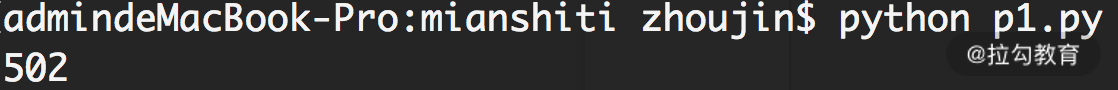【数学角度解答】

P(A>B)=P(M+N>B)= P(N=0)·P(M+0>B)+P(N=1)·P(M+1>B)= 0.5·P(M+0>B) + 0.5·P(M+1>B)

例题2 数据上溢问题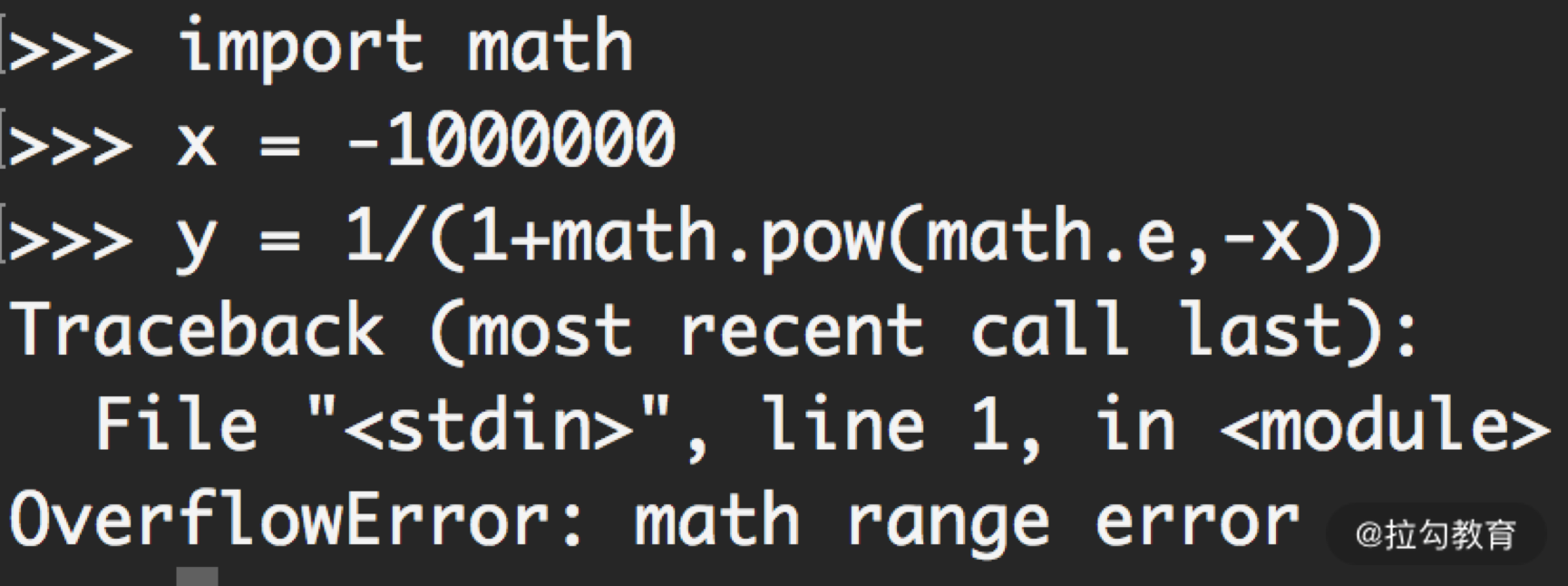y = 1/(1+e-x) = ex/(ex+1)。

• 如果 x>0，则 y = 1/(1+e-x)
• 如果 x<0，则 y = ex/(1+ex)

import math

def sigmoid(x):

if x < 0:

y = math.pow(math.e,x) / (1 + math.pow(math.e,x))

else:

y = 1 / (1 + math.pow(math.e,-x))

return y

a = -1000000

b = 1000000

print sigmoid(a)

print sigmoid(b)

• 在 Sigmoid 函数的代码中，第 4 行，判断 x 和 0 的大小关系；
• 如果 x 为负数，则通过第 5 行的公式计算 y；
• 如果 x 不是负数，则通过第 7 行的公式来计算 y。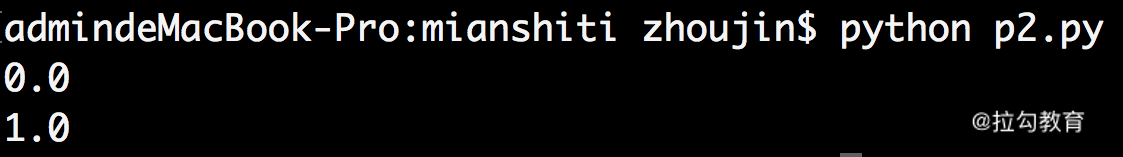例题3 投点距离期望问题

import random

import math

inCircle = 0

distance = 0.0

for _ in range(1000):

x = 1.0 * random.randint(0,1000) / 100

y = 1.0 * random.randint(0,1000) / 100

if x * x + y * y > 100:

continue

else:

inCircle += 1

distance += math.sqrt(x * x + y * y)

print distance / inCircle

• 第 4 行，保存合法的投射次数变量；
• 第 5 行，是累计的距离之和变量；
• 第 6 行，通过 for 循环执行多次的投射动作；
• 每次投射，第 7 行和第 8 行，随机地生成投射点的坐标变量 x 和 y（在这里，我们精确到小数点后两位）；
• 第 9 行，如果坐标点的平方和超过 100，也就是投射点在 10 厘米的圆形之外；
• 那么第 10 行，执行 continue，继续下一轮循环；
• 否则，说明投射点在圆内，执行第 11 行的代码；
• 第 12 行，合法投射次数加 1；
• 第 13 行，通过本次投射点到圆心的距离，更新累计的距离之和；
• 最后第 14 行，打印累计距离和合法投射次数的比值，得到了平均距离。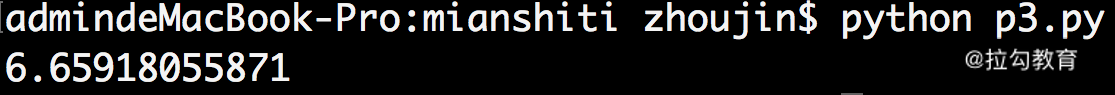【数学角度解答】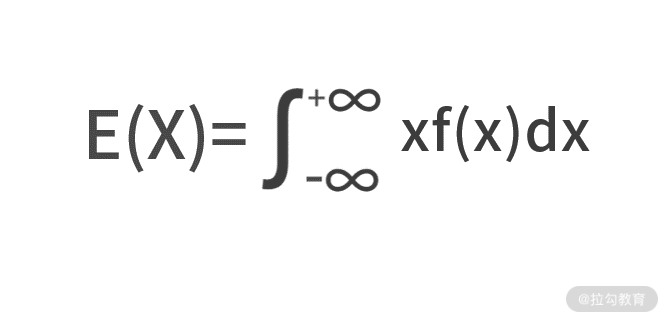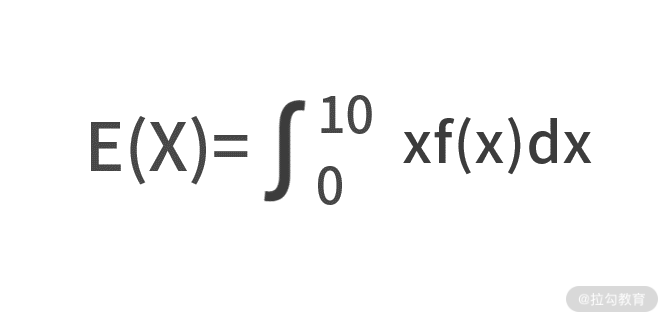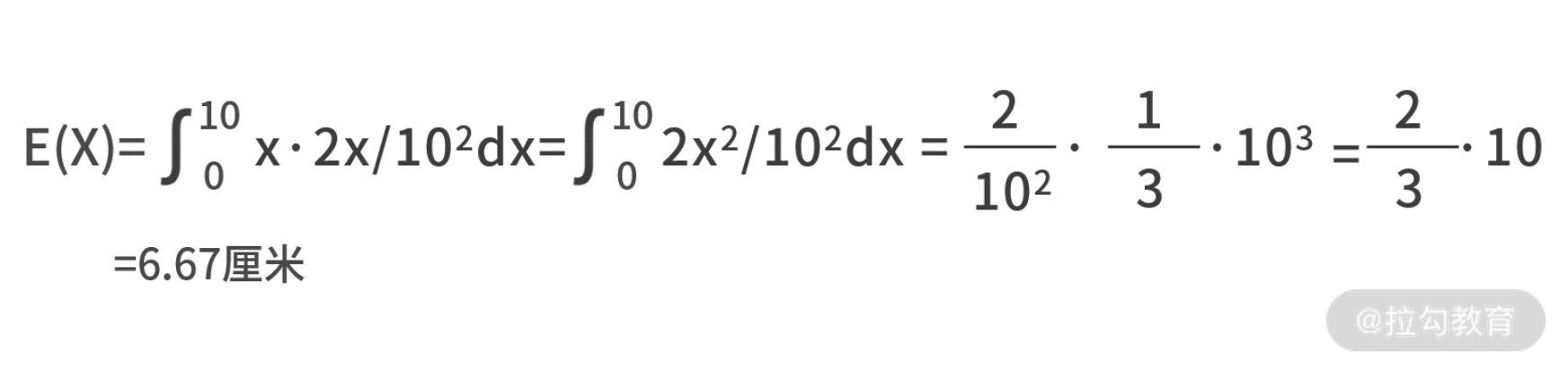小结

• 第一个角度，从问题出发去寻找突破口。

• 第二个角度，从已知条件出发去寻找突破口。